正交各向异性带型中裂纹诱导的温度场分析Analysis of the Temperature Field Induced by a Crack in an Orthotropic Strip

• 全文下载: PDF(675KB)    PP.439-447   DOI: 10.12677/APP.2018.810056
• 下载量: 166  浏览量: 264   科研立项经费支持

This paper investigates the problem of a crack embedded in an orthotropic strip under a thermal loading. Using the Fourier transform, the thermal boundary value problem is transformed into a singular integral equation. The Lobatto-Chebyshev formula is used to further derive the linear system of algebraic equations. Through the numerical calculation, the temperature jump across the crack surface is obtained. Finally, the influence of crack position and crack size on the temperature field is reflected in the form of a graph.

1. 引言

2. 研究对象

${q}_{x}=-{\lambda }_{x}\frac{\partial \theta }{\partial x}$${q}_{y}=-{\lambda }_{y}\frac{\partial \theta }{\partial y},$

$\frac{\partial {q}_{x}}{\partial x}+\frac{\partial {q}_{y}}{\partial y}=0$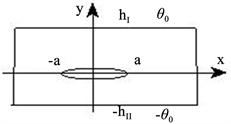Figure 1. A crack in an orthotropic strip

${\lambda }^{2}\frac{{\partial }^{2}\theta }{\partial {x}^{2}}+\frac{{\partial }^{2}\theta }{\partial {y}^{2}}=0,$ (1)

${\theta }^{\left(I\right)}\left(x,{h}_{I}\right)={\theta }_{0},{\theta }^{\left(II\right)}\left(x,-{h}_{II}\right)=-{\theta }_{0},\text{\hspace{0.17em}}x>0,$ (2)

${\theta }^{\left(I\right)}\left(x,0\right)={\theta }^{\left(II\right)}\left(x,0\right),\text{\hspace{0.17em}}x>a,$ (3)

${q}_{y}^{\left(I\right)}\left(x,0\right)={q}_{y}^{\left(II\right)}\left(x,0\right),\text{\hspace{0.17em}}x>a,$ (4)

${q}_{y}^{\left(I\right)}\left(x,0\right)={q}_{y}^{\left(II\right)}\left(x,0\right)={q}_{c},\text{\hspace{0.17em}}0 (5)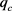是热流密度， ${q}_{x},{q}_{y}$ 分别是 $x$ 轴和 $y$ 轴方向的热流分量， $\theta$ 是温度，而 $\lambda ,{\lambda }_{x},{\lambda }_{y}$ 是方程热传导系数，它们具有重要的物理意义。

3. 求解过程

$\stackrel{¯}{f}\left(\xi \right)=\frac{2}{\text{π}}{\int }_{0}^{+\infty }f\left(x\right)\mathrm{cos}\left(\xi x\right)\text{\hspace{0.17em}}\text{d}x,$

$f\left(x\right)={\int }_{0}^{+\infty }\stackrel{¯}{f}\left(\xi \right)\mathrm{cos}\left(\xi x\right)\text{d}\xi .$

${f}^{\left(n\right)}\left(x\right)={\left(-i\xi \right)}^{n}\stackrel{¯}{f}\left(\xi \right).$

$\frac{{\text{d}}^{2}f}{\text{d}{y}^{2}}+p\frac{\text{d}f}{\text{d}y}+qf=0,$ (6)

${m}^{2}+pm+q=0.$

$f={c}_{1}{e}^{{m}_{1}y}+{c}_{2}{e}^{{m}_{2}y},$ (7)

$f={c}_{1}{e}^{{m}_{1}y}+{c}_{2}y{e}^{{m}_{1}y},$ (8)

${e}^{x}=\mathrm{sinh}\left(x\right)+\mathrm{cosh}\left(x\right),$

$f=\underset{i=1}{\overset{2}{\sum }}{c}_{i}\left[\mathrm{cosh}\left({m}_{i}y\right)+\mathrm{sinh}\left({m}_{i}y\right)\right],$ (9)

$f=\left({c}_{1}+{c}_{2}y\right)\left[\mathrm{cosh}\left({m}_{1}y\right)+\mathrm{sinh}\left({m}_{1}y\right)\right].$ (10)

3.1. 温度场的求解

${\theta }_{1}^{\left(j\right)}\left(x,y\right)=\frac{2}{\text{π}}{\int }_{0}^{\infty }\left[{A}^{\left(j\right)}\left(\xi \right)\mathrm{cosh}\left(\lambda \xi y\right)+{B}^{\left(j\right)}\left(\xi \right)\mathrm{sinh}\left(\lambda \xi y\right)\right]\mathrm{cos}\left(\xi x\right)\text{d}\xi ,$

$\frac{{\partial }^{2}{\theta }_{2}\left(y\right)}{\partial y}=0,$ (11)

${\theta }_{2}\left({h}_{I}\right)={\theta }_{0},$ (12)

${\theta }_{2}\left(-{h}_{II}\right)=-\theta$ (13)

${\theta }_{2}\left(y\right)={A}_{0}y+{B}_{0},$

${A}_{0}=\frac{2{\theta }_{0}}{{h}_{I}+{h}_{II}},\text{\hspace{0.17em}}{B}_{0}=\frac{{\theta }_{0}\left({h}_{II}-{h}_{I}\right)}{{h}_{I}+{h}_{II}}.$

${\theta }^{\left(j\right)}\left(x,y\right)=\frac{2}{\text{π}}{\int }_{0}^{\infty }\left[{A}^{\left(j\right)}\left(\xi \right)\mathrm{cosh}\left(\lambda \xi y\right)+{B}^{\left(j\right)}\left(\xi \right)\mathrm{sinh}\left(\lambda \xi y\right)\right]\mathrm{cos}\left(\xi x\right)\text{d}\xi +{A}_{0}y+{B}_{0}.$ (14)

${B}^{\left(j\right)}\left(\xi \right)={\delta }^{\left\{j\right\}}\mathrm{coth}\left(\lambda \xi {h}_{i}\right){A}^{\left(j\right)}\left(\xi \right),$

${\theta }^{\left(j\right)}\left(x,y\right)=\frac{2}{\text{π}}{\int }_{0}^{\infty }\frac{\mathrm{sinh}\left[\lambda \xi \left({h}_{j}+{\delta }^{\left\{j\right\}}y\right)\right]}{\mathrm{sinh}\left(\lambda \xi {h}_{j}\right)}{A}^{\left(j\right)}\left(\xi \right)\mathrm{cos}\left(\xi x\right)\text{d}\xi +{A}_{0}y+{B}_{0},$ (15)

${q}_{y}^{\left(j\right)}\left(x,y\right)=-{\lambda }_{y}{\delta }^{\left\{j\right\}}\frac{2}{\text{π}}{\int }_{0}^{\infty }\frac{\mathrm{cosh}\left[\lambda \xi \left({h}_{j}+{\delta }^{\left\{j\right\}}y\right)\right]}{\mathrm{sinh}\left(\lambda \xi {h}_{j}\right)}{A}^{\left(j\right)}\left(\xi \right)\mathrm{cos}\left(\xi x\right)\text{d}\xi -{\lambda }_{y}{A}_{0}.$ (16)

${\phi }_{1}\left(x\right)=\frac{\partial \left[{\theta }^{\left(I\right)}\left(x,0\right)-{\theta }^{\left(II\right)}\left(x,0\right)\right]}{\partial x},$ (17)

${\theta }^{\left(I\right)}\left(x,0\right)=\frac{2}{\text{π}}{\int }_{0}^{\infty }{A}^{\left(I\right)}\left(\xi \right)\mathrm{cos}\left(\xi x\right)\text{d}\xi +{B}_{0},$ (18)

${\theta }^{\left(II\right)}\left(x,0\right)=\frac{2}{\text{π}}{\int }_{0}^{\infty }{A}^{\left(II\right)}\left(\xi \right)\mathrm{cos}\left(\xi x\right)\text{d}\xi +{B}_{0},$ (19)

${A}^{\left(I\right)}\left(\xi \right)-{A}^{\left(II\right)}\left(\xi \right)=-\frac{1}{\xi }{\int }_{0}^{a}{\phi }_{1}\left(s\right)\mathrm{sin}\left(\xi s\right)\text{d}s.$ (20)

${A}^{\left(II\right)}\left(\xi \right)=-\frac{\mathrm{coth}\left(\lambda \xi {h}_{I}\right)}{\mathrm{coth}\left(\lambda \xi {h}_{II}\right)}{A}^{\left(I\right)}\left(\xi \right),$ (21)

${A}^{\left(I\right)}\left(\xi \right)=-\frac{1}{\xi }{\int }_{0}^{a}\frac{\mathrm{coth}\left(\lambda \xi {h}_{II}\right)}{\mathrm{coth}\left(\lambda \xi {h}_{I}\right)+\mathrm{coth}\left(\lambda \xi {h}_{II}\right)}{\phi }_{1}\left(s\right)\mathrm{sin}\left(\xi s\right)\text{d}s,$ (22)

${A}^{\left(II\right)}\left(\xi \right)=\frac{1}{\xi }{\int }_{0}^{a}\frac{\mathrm{coth}\left(\lambda \xi {h}_{I}\right)}{\mathrm{coth}\left(\lambda \xi {h}_{I}\right)+\mathrm{coth}\left(\lambda \xi {h}_{II}\right)}{\phi }_{1}\left(s\right)\mathrm{sin}\left(\xi s\right)\text{d}s,$ (23)

${\theta }^{\left(I\right)}\left(x,0\right)=-\frac{\text{2}}{\text{π}}{\int }_{0}^{a}\left[{\int }_{0}^{\infty }\frac{1}{\xi }\frac{\mathrm{coth}\left(\lambda \xi {h}_{II}\right)}{\mathrm{coth}\left(\lambda \xi {h}_{I}\right)+\mathrm{coth}\left(\lambda \xi {h}_{II}\right)}\mathrm{sin}\left(\xi s\right)\mathrm{cos}\left(\xi x\right)\text{d}\xi \right]\text{\hspace{0.17em}}{\phi }_{1}\left(s\right)\text{d}s+{B}_{0},$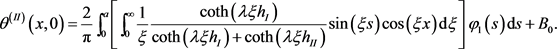$\frac{2}{\text{π}}{\int }_{0}^{a}\left[{\int }_{0}^{\infty }\frac{1}{\xi }\frac{{\lambda }_{y}\lambda \xi \mathrm{coth}\left(\lambda \xi {h}_{I}\right)\mathrm{coth}\left(\lambda \xi {h}_{II}\right)}{\mathrm{coth}\left(\lambda \xi {h}_{I}\right)+\mathrm{coth}\left(\lambda \xi {h}_{II}\right)}\mathrm{sin}\left(\xi s\right)\mathrm{cos}\left(\xi x\right)\text{d}\xi \right]\text{\hspace{0.17em}}{\phi }_{1}\left(s\right)\text{d}s=-\left({q}_{c}+{\lambda }_{y}{A}_{0}\right).$ (24)

3.2. 奇异积分方程的求解

$M\left(\xi \right)=\frac{{\lambda }_{y}\lambda \xi \mathrm{coth}\left(\lambda \xi {h}_{I}\right)\mathrm{coth}\left(\lambda \xi {h}_{II}\right)}{\mathrm{coth}\left(\lambda \xi {h}_{I}\right)+\mathrm{coth}\left(\lambda \xi {h}_{II}\right)}$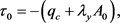$\frac{2}{\text{π}}{\int }_{0}^{a}\left[{\int }_{0}^{\infty }\frac{M\left(\xi \right)}{\xi }\mathrm{sin}\left(\xi s\right)\mathrm{cos}\left(\xi x\right)\text{d}\xi \right]\text{\hspace{0.17em}}{\phi }_{1}\left(s\right)\text{d}s={\tau }_{0}.$ (25)

$\frac{2}{\text{π}}{\int }_{0}^{\infty }\mathrm{sin}\left(\xi s\right)\mathrm{cos}\left(\xi x\right)\text{d}\xi =\frac{1}{\text{π}}\left(\frac{1}{s-x}+\frac{1}{s+x}\right),$

$\Lambda =\underset{x\to +\infty }{\mathrm{lim}}\frac{M\left(\xi \right)}{\xi },$

$\Lambda$ 是由材料性质决定的常数，可以通过数值计算解得。所以方程(25)可化为以下奇异积分方程

$\frac{1}{\text{π}}{\int }_{-a}^{a}\frac{\Lambda }{s-x}{\phi }_{1}\left(s\right)\text{d}s+\frac{1}{\text{π}}{\int }_{-a}^{a}k\left(s,x\right){\phi }_{1}\left(s\right)\text{d}s={\tau }_{0},$ (26)

$k\left(s,x\right)={\int }_{0}^{\infty }\left[\frac{M\left(\xi \right)}{\xi }-\Lambda \right]\mathrm{sin}\left(\xi s\right)\mathrm{cos}\left(\xi x\right)\text{d}\xi .$

$x=a\stackrel{¯}{x}$$s=a\stackrel{¯}{s}$${\phi }_{1}\left(s\right)=\frac{Y\left(\stackrel{¯}{s}\right)}{\sqrt{1-{\stackrel{¯}{s}}^{2}}},$

$\frac{1}{\text{π}}{\int }_{-1}^{1}\frac{\Lambda }{\stackrel{¯}{s}-\stackrel{¯}{x}}\frac{Y\left(\stackrel{¯}{s}\right)}{\sqrt{1-{\stackrel{¯}{s}}^{2}}}\text{d}\stackrel{¯}{s}+\frac{1}{\text{π}}{\int }_{-1}^{1}\stackrel{¯}{k}\left(\stackrel{¯}{s},\stackrel{¯}{x}\right)\frac{Y\left(\stackrel{¯}{s}\right)}{\sqrt{1-{\stackrel{¯}{s}}^{2}}}\text{\hspace{0.17em}}\text{d}\stackrel{¯}{s}={\tau }_{0},$ (27)

$\stackrel{¯}{k}\left(\stackrel{¯}{s},\stackrel{¯}{x}\right)=a{\int }_{0}^{\infty }\left[\frac{M\left(\xi \right)}{\xi }-\Lambda \right]\mathrm{sin}\left(\xi a\stackrel{¯}{s}\right)\mathrm{cos}\left(\xi a\stackrel{¯}{x}\right)\text{d}\xi .$

${\int }_{-1}^{1}{\phi }_{1}\left(x\right)\text{d}x=0.$ (28)

Lobatto-Chebyshev积分公式，获得以下代数方程组

$\frac{1}{L}\underset{l=0}{\overset{L}{\sum }}\frac{{\lambda }_{l}\Lambda }{{\stackrel{¯}{s}}_{l}-{\stackrel{¯}{x}}_{m}}Y\left({\stackrel{¯}{s}}_{l}\right)+\frac{1}{L}\underset{l=0}{\overset{L}{\sum }}{\lambda }_{l}\stackrel{¯}{k}\left(\stackrel{¯}{s},\stackrel{¯}{x}\right)Y\left({\stackrel{¯}{s}}_{l}\right)={\tau }_{0},$ (29)

$\underset{l=0}{\overset{L}{\sum }}{\lambda }_{l}Y\left({\stackrel{¯}{s}}_{l}\right)=0,$ (30)

${\stackrel{¯}{x}}_{m}=\mathrm{cos}\left[\left(2m-1\right)\text{π}/\left(2L\right)\right]$$m=1,2,\cdots ,L,$

${\stackrel{¯}{s}}_{l}=\mathrm{cos}\left(l\text{π}/L\right)$$l=0,1,2,\cdots ,L,$

${\lambda }_{0}={\lambda }_{L}=1/2$${\lambda }_{1}=\cdots ={\lambda }_{L-1}=1.$

$\begin{array}{c}{\int }_{x}^{a}{\phi }_{1}\left(s\right)\text{d}s={\int }_{x}^{a}\frac{\partial \left[{\theta }^{\left(I\right)}\left(s,0\right)-{\theta }^{\left(II\right)}\left(s,0\right)\right]}{\partial s}\text{d}s\\ =\left[{\theta }^{\left(I\right)}\left(s,0\right)-{\theta }^{\left(II\right)}\left(s,0\right)\right]|\begin{array}{c}a\\ x\end{array}\\ =-\left[{\theta }^{\left(I\right)}\left(x,0\right)-{\theta }^{\left(II\right)}\left(x,0\right)\right],\end{array}$

${\theta }^{\left(I\right)}\left(x,0\right)-{\theta }^{\left(II\right)}\left(x,0\right)=-a{\int }_{\stackrel{¯}{x}}^{1}\frac{Y\left(\stackrel{¯}{s}\right)}{\sqrt{1-{\stackrel{¯}{s}}^{2}}}\text{\hspace{0.17em}}\text{d}\stackrel{¯}{s}.$

${\theta }^{\left(I\right)}\left(x,0\right)-{\theta }^{\left(II\right)}\left(x,0\right)\approx -a\underset{l=0}{\overset{L/2}{\sum }}{\lambda }_{l}Y\left({\stackrel{¯}{s}}_{l}\right).$ (31)

4. 数值实例

5. 结论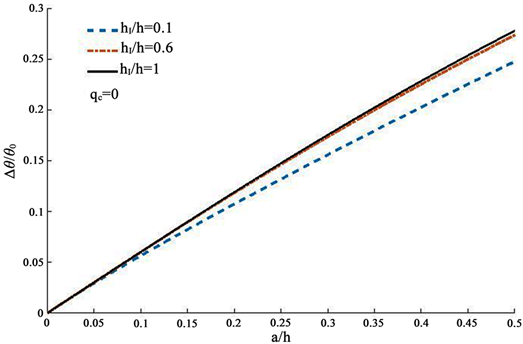Figure 2. The influences of crack location and size on $\Delta \theta /{\theta }_{0}$

  赵建生. 断裂力学及断裂物理[M]. 华中科技大学出版社, 2003.  范天佑. 断裂理论基础[M]. 北京: 科学出版社, 2003.  余寿文. 断裂力学的历史发展与思考[J]. 力学与实践, 2015, 37(3): 390-394.  Chen, B.X. and Zhang, X. (1988) Thermoelasticity Problem of an Orthotropic Plate with Two Collinear Cracks. International Journal of Fracture, 38, 161-192.  Zhong, X.C. and Kang, Y.L. (2012) A Thermal-Medium Crack Model. Mechanics of Materials, 51, 110-117. https://doi.org/10.1016/j.mechmat.2012.04.013  Zhong, X.C., Wu, B. and Zhang, K.S. (2013) Thermally Conducting Col-linear Cracks Engulfed by Thermomechanical Field in a Material with Orthotropy. Theoretical and Applied Fracture Mechanics, 65, 61-68. https://doi.org/10.1016/j.tafmec.2013.05.009  Tsai, Y.M. (1984) Orthotropic Thermoelastic Problem of Uniform Heat Flow Disturbed by a Central Crack. Journal of Composite Materials, 18, 122-131. https://doi.org/10.1177/002199838401800203  Chen, J., Soh, A.K., Liu, J., et al. (2004) Thermal Fracture Analysis of a Functionally Graded Orthotropic Strip with a Crack. International Journal of Mechanics & Materials in Design, 1, 131-141. https://doi.org/10.1007/s10999-004-1489-9  Li, X.F. and Duan, X.Y. (2001) Closed-Form Solution for a Mode-III Crack at the Mid-Plane of a Piezoelectric Layer. Mechanics Research Communications, 28, 703-710. https://doi.org/10.1016/S0093-6413(02)00222-7  Itou, S. (2000) Thermal Stress Intensity Factors of an Infinite Orthotropic Layer with a Crack. International Journal of Fracture, 103, 279-291. https://doi.org/10.1023/A:1007630808737  顾樵. 数学物理方法[M]. 北京: 科学出版社, 2012.  Masjed-Jamei, M., et al. (2005) The First Kind Chebyshev CLobatto Quadrature Rule and Its Numerical Improvement. Applied Mathematics and Computation, 171, 1104-1118. https://doi.org/10.1016/j.amc.2005.01.132  Chen, J. (2005) Determination of Thermal Stress Intensity Factors for an Interface Crack in a Graded Orthotropic Coating-Substrate Structure. International Journal of Fracture, 133, 303-328. https://doi.org/10.1007/s10704-005-4728-7  路见可, 杜金元. 奇异积分方程的数值解法[J]. 数学进展, 1991(3): 278-293.  Gradshteyn, I.S. and Ryzhik, I.M. (2007) In Table of Integrals, Series, and Products. Mathematics of Computation, 20, 1157-1160.